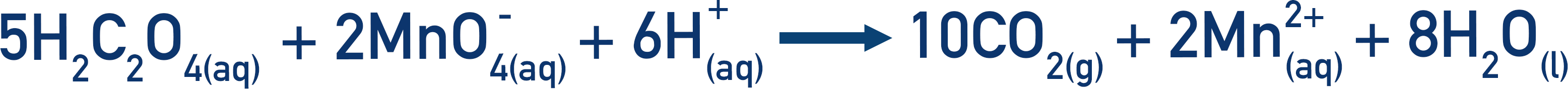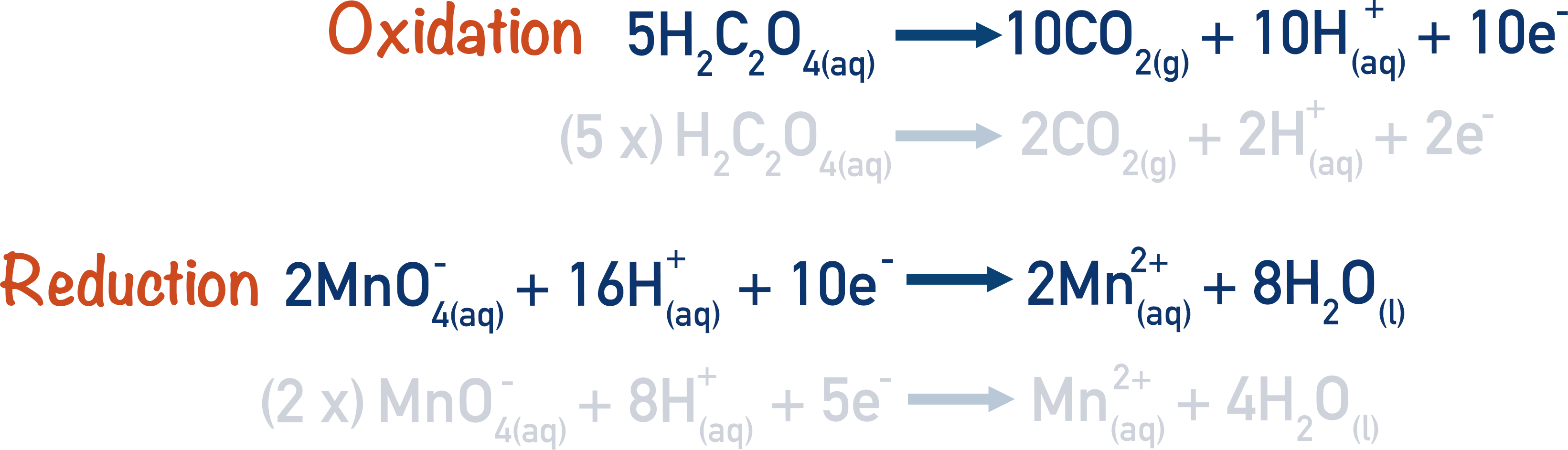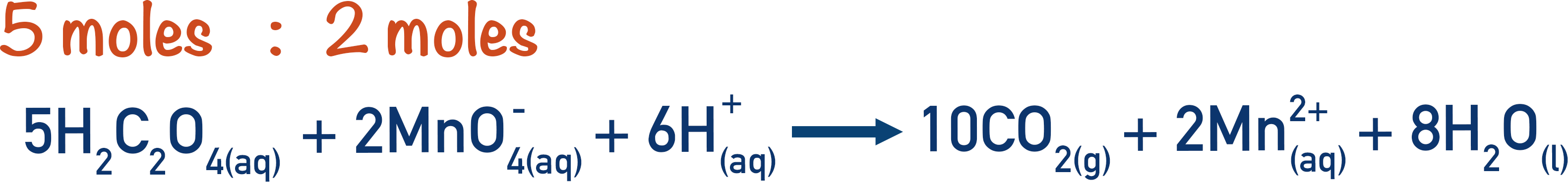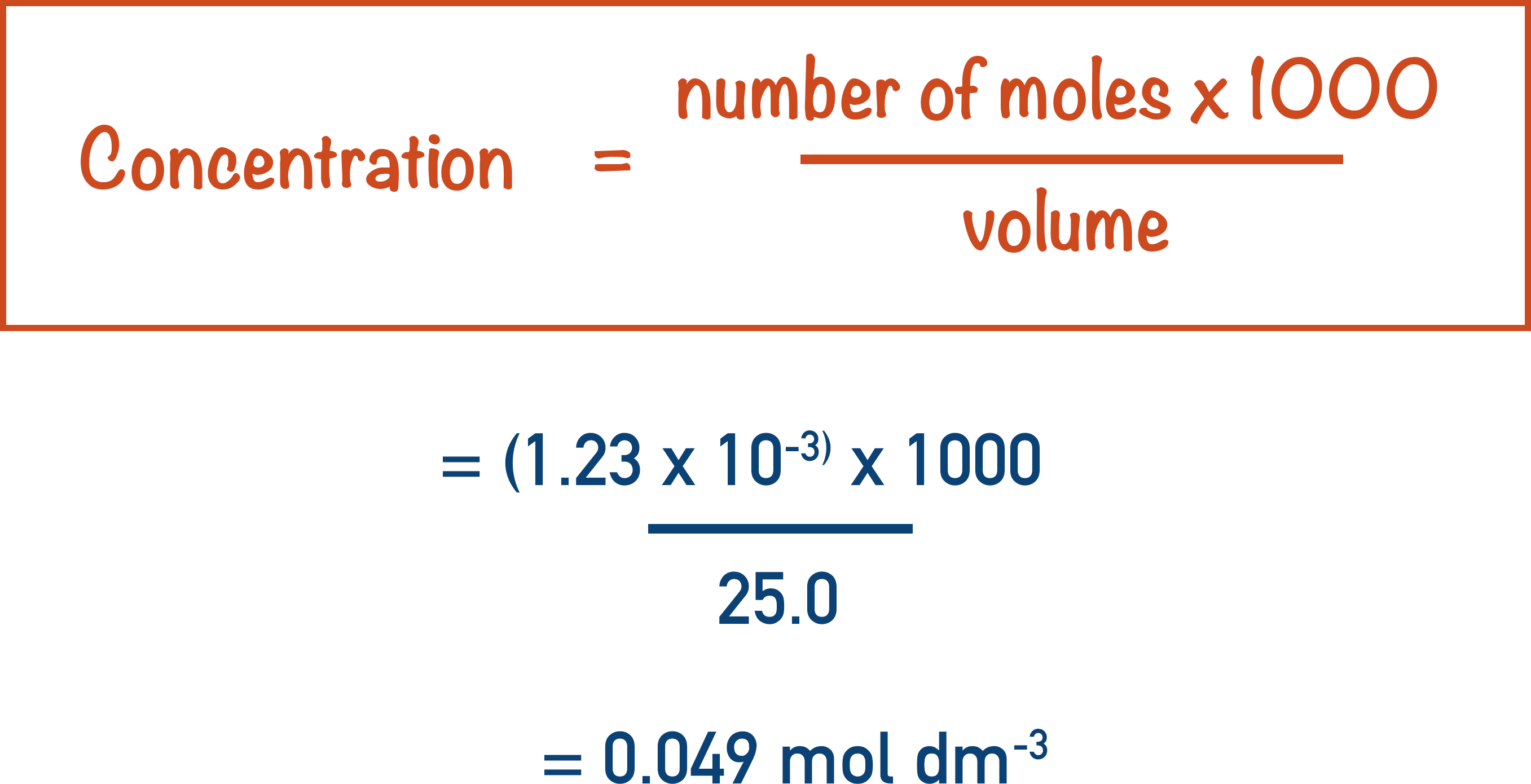Quick Notes Redox Titrations

• Titrations can be used to find the unknown concentration of a solution.
• Redox reactions consist of a species that is oxidised and a species that is reduced.
• If the moles of one species is known, using the ratio of the oxidised species to the reduced species, the moles of the other species can be found and the concentration calculated.

Full Notes Redox Titrations

Redox reactions describe reactions where one species gains electrons (reduction) and another species loses electrons (oxidation). As transition elements have multiple oxidation states, they can form different charged ions and often take part in redox reactions.

Redox titrations use redox reactions to find the concentration of a species or molecule – in the same way titrations are used to find the find the concentration of an acid or alkali (see Titrations). An oxidising agent and a reducing agent react, and when a colour change occurs, a set amount of either one has reacted or been produced.

Redox titrations can look complicated, but if approached in the right way they can be tackled confidently. Determining the redox reaction(s) taking place is always the first step. This is the part that frequently causes students alarm.

Half-equations are used to show the species being oxidised and reduced.

For example, the reaction between ethanedioic acid and potassium manganate (VII) solution, can be written as:This is a redox reaction, the ethanedioic acid is oxidised and the manganate ions are reduced. Now, we can write out the half-equations that show this oxidation and reduction.By using these half-equations and the overall equation for the reaction, we can find the concentration of one of the reactants with a titration.

For example:exam-style

In a titration, 24.5 cm3 of 0.02 mol dm-3 potassium manganate (VII) solution was required to react completely with 25.0cm3 of ethanedioic acid solution.

Calculate the concentration of the ethanoic acid solution.

The first thing to do is find the number of moles of manganate ions that reacted.From the overall equation, the ratio of manganate ions to ethanedioic acid is 2:5. This means that for every two moles of manganate ions, five moles of ethanedioic acid are required.We can use this ratio to calculate the moles of ethanedioic acid that must have reacted with the 4.9x10-4 moles of manganate ions.We know the volume of ethanedioic acid that was used in the titration was 25.0cm3. By re-arranging the equation used previously, we can find the concentration of this solution.